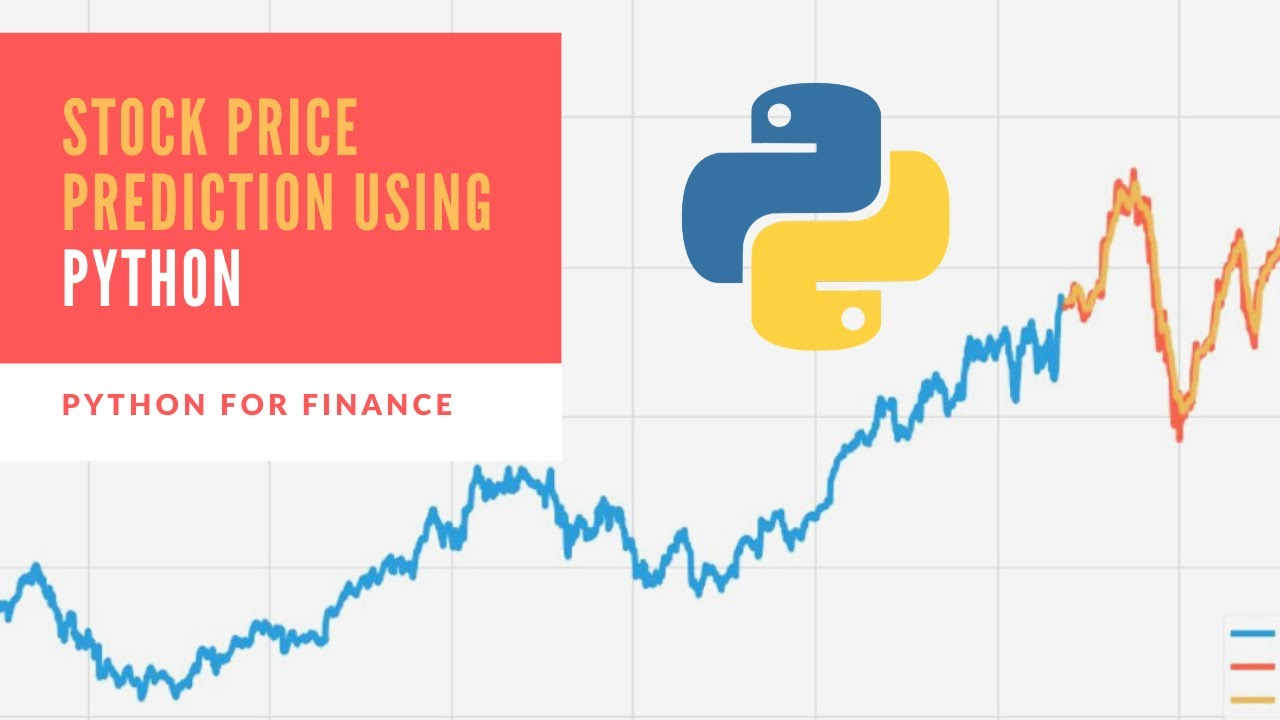# Stock Price Prediction Using Python & Machine LearningStock Price Prediction Using Python & Machine Learning (LSTM). In this video you will learn how to create an artificial neural network called Long Short Term Memory to predict the future price of stock.

Stock Price Prediction Using Python & Machine Learning (LSTM).
In this video you will learn how to create an artificial neural network called Long Short Term Memory to predict the future price of stock.

NOTE: In the video to calculate the RMSE I put the following statement:
rmse=np.sqrt(np.mean((predictions- y_test)**2))

When in fact I meant to put :
rmse=np.sqrt(np.mean(((predictions- y_test)**2)))

You can use the following statements to calculate RMSE:

1. rmse =np.sqrt(np.mean(((predictions- y_test)**2)))
2. rmse = np.sqrt(np.mean(np.power((np.array(y_test)-np.array(predictions)),2)))
3. rmse = np.sqrt(((predictions - y_test) ** 2).mean())